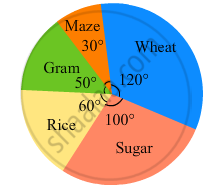Advertisement Remove all ads

# The Pie-chart Given in the Following Shows the Annual Agricultural Production of an Indian State. If the Total Production of All the Commodities is 81000 Tonnes, Find the Production (In Tonnes) of - Mathematics

Sum

The pie-chart given in the following shows the annual agricultural production of an Indian state. If the total production of all the commodities is 81000 tonnes, find the production (in tonnes) of
(i) Wheat
(ii) Sugar
(iii) Rice
(iv) Maize
(v) GramAdvertisement Remove all ads

#### Solution

(i) $\text{ Production of wheat }= \frac{\text{ Central angle for wheat }\times\text{ Total production }}{360^\circ}$
$= \frac{120^\circ \times 81000}{360^\circ} = 27000\text{ tonnes }$

(ii)$\text{ Production of sugar }= \frac{\text{ Central angle for sugar }\times\text{ Total production }}{360^\circ}$
$= \frac{10^\circ \times 81000}{360^\circ} = 22500\text{ tonnes }$

(iii) $\text{ Production of rice }= \frac{\text{ Central angle for Rice }\times\text{ Total production }}{360^\circ}$
$= \frac{60^\circ \times 81000}{360^\circ} = 13500\text{ tonnes }$

(iv) $\text{ Production of maize }= \frac{\text{ Central angle for maize }\times\text{ Total production }}{360^\circ}$
$= \frac{30^\circ \times 81000}{360^\circ} = 6750\text{ tonnes }$

(v) $\text{ Production of gram }= \frac{\text{ Central angle for Gram }\times\text{ Total production }}{360^\circ}$
$= \frac{120^\circ \times 81000}{360^\circ} = 11250\text{ tonnes }$

Is there an error in this question or solution?
Advertisement Remove all ads

#### APPEARS IN

RD Sharma Class 8 Maths
Chapter 25 Data Handling-III (Pictorial Representation of Data as Pie Charts or Circle Graphs)
Exercise 25.2 | Q 2 | Page 21
Advertisement Remove all ads

#### Video TutorialsVIEW ALL 

Advertisement Remove all ads
Share
Notifications

View all notifications

Forgot password?